Here’s something to get excited about: Google just launched a new workflow automation tool!

Google Tables is a tool for teams and businesses that combines the flexibility of a spreadsheet with the power of a database.

Best of all, it provides a more visual way to present information than a spreadsheet.

There are so many ways to use this tool, and I’ll show some of them later in this post. I could see teams and individuals using it to organize and track projects for both work and home life, similar to how many people already use tools like Trello, Asana or Airtable — and yes, there’s even a kanban view!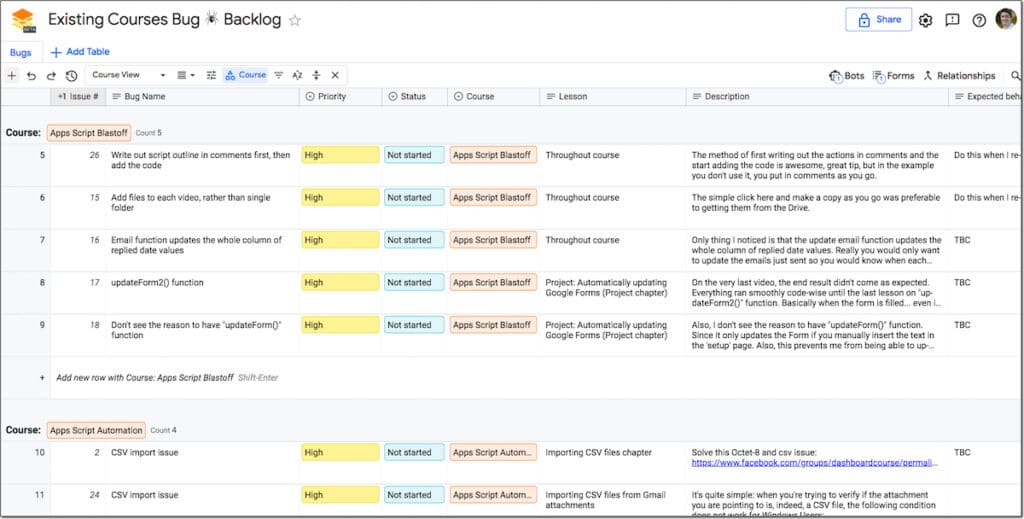Then you can group and link these tables into Workspaces to create process workflows:

Finally, sprinkle them with automation magic to save yourself time, using customizable, no-code Bots:

But even if you love spreadsheets as much as I do, they’re not suitable for everything.

We’re all guilty of using spreadsheets to do things they’re not designed for.

For example, they’re not the best tool for managing workflows and automating multi-step processes. Spreadsheets set up like that often end up being complex and unwieldy to use.

Those workflows we track with spreadsheets — managing events, onboarding new hires, managing complex projects, etc. — are better suited to managing with this new Google Tables tool.

Tables are the fundamental construct of the Google Tables product. They’re containers that hold structured data, i.e. ordered data recorded in rows.

Workspaces are collections of Tables grouped together. Tables can belong to multiple workspaces. When you open a workspace, you open all the Tables included in that workspace.

Columns in each Table are strongly-typed, meaning the data type you store in that column is predefined when you select the column type. This is different from a spreadsheet where you can store any type of data in any cell (unless you have data validation in place).

Views are saved versions of a Table with the data shown in a specific way. You can have multiple saved versions of a single Table, for example with different filters applied.

## How much does Google Tables cost?

Google Tables is generally available to anyone with a Google account in the US at the moment.

Every country has different rules and norms around data privacy etc. so the team is starting in the US and will expand around the world in time. If you’re outside the US, you can express your interest via this form.

It’s currently a beta version, which means the product is still evolving and improving.

Free and paid tiers are available.

The paid tier costs \$10/month and gives you additional storage, more tables and more bot (automation) actions. There’s a 3-month free trial of the paid tier, so you can try out all the features.

## How I Use Google Tables

I’ve had access to the alpha version of Tables for the past 6 months. It’s quickly become an indispensable tool for the day-to-day running of my business.

I use it for two major workflows at the moment:

1. My weekly planner
2. An issue tracker for my courses

I also plan to move several other workflows from Google Sheets into Tables in the near future: my site content planning / SEO spreadsheet, my newsletter tracker, and my business process directory.

### Workflow 1: Weekly Planner Kanban Board View

For years I used Trello’s kanban board (card) layout to manage my business week-to-week tasks.

Now I use Google Tables to do that.

I use it as a sort of rolling 7-day calendar, but I prefer it to a calendar because of the flexibility it affords.

Ultimately, it’s a combination of Trello (kanban board) + Tasks (To-Do list) + Calendar (events).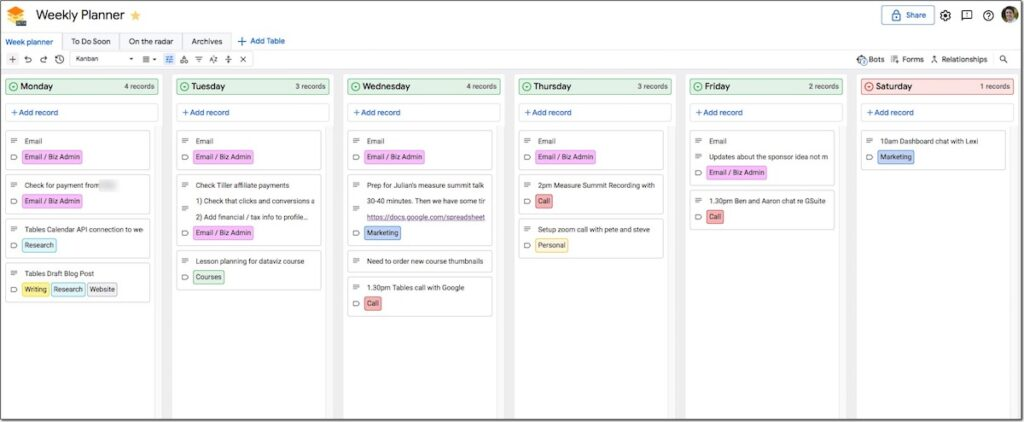Zooming in a little, here’s an example of my tasks for a given day: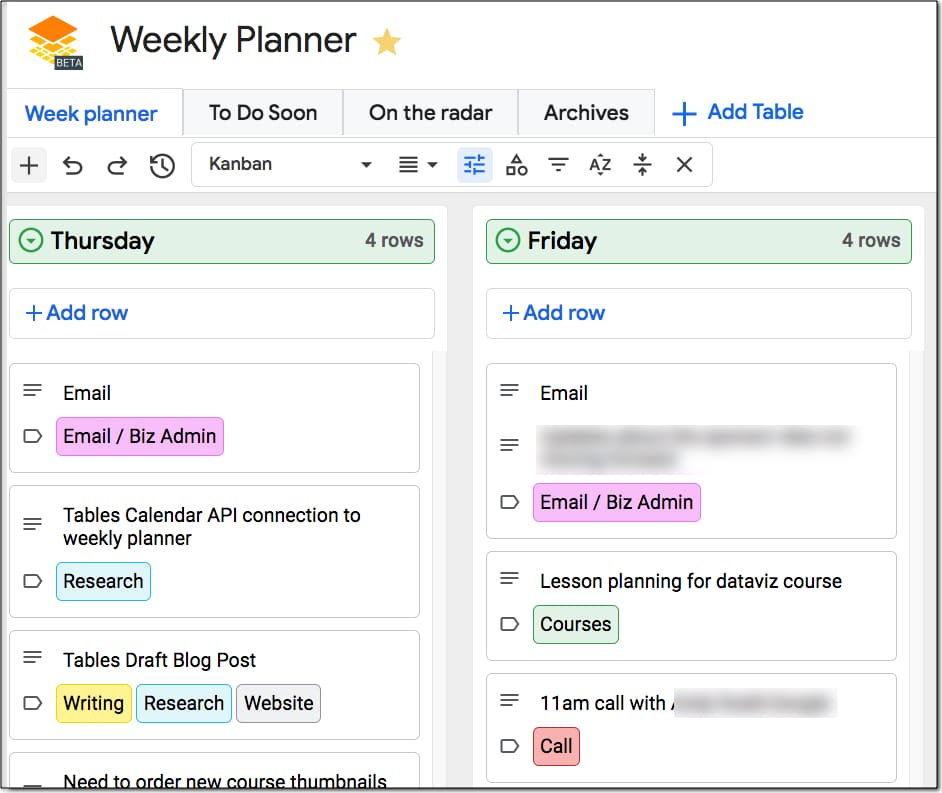Each record is a row of data in a Table, presented in the kanban board view. I can drag records to move tasks to a different day. I can easily add new tasks or notes, and I can archive tasks when I complete them, using a bot.

#### Automation With Bots

Bots are automations that carry out a predefined set of instructions. In Tables, bots are created without writing any code.

In this weekly planner, I use them to move records from one Table to another.

For example, I like to archive tasks when I complete them.

I check an archive checkbox and then a bot moves the record into the Archive table.

You can do lots of other things with bots too.

They can be triggered when something happens (e.g. a record gets added), on a set schedule (daily or weekly) or even by another bot.

They can perform actions like modifying records, adding records, sending emails or pinging webhooks (to send a chat notification to Slack for example).

#### Accessing Tables With Apps Script

And yes, whilst we’re on the subject of automation, Tables has an API and is also accessible programmatically via Apps Script!

(Here’s a Google Apps Script explainer if you haven’t used it before.)

For Apps Script, you must first enable the Tables API under the Advanced Service menu. Then you can access Tables by the Table ID, found after the /table/ part of the URL.

A basic Apps Script code to get the Table rows looks like this:

```var tableName = "tables/XXXXXXXXXXXXXXXX";
var tableRows = Area120Tables.Tables.Rows.list(tableName).rows;```

### Workflow 2: Issue Tracker For My Online Courses

The other workflow I’ve setup in Tables is an issue tracker for my online courses.

Whenever someone contacts me with an issue on one of my courses, I log it in this Table, with tags to indicate which course, how urgent it is, where I’m up to etc.

It’s much easier to organize and see the issues compared to a plain data table in a spreadsheet. It requires a lot less effort to view the information.

Here’s an example of the issue tracker in a simple Google Sheet: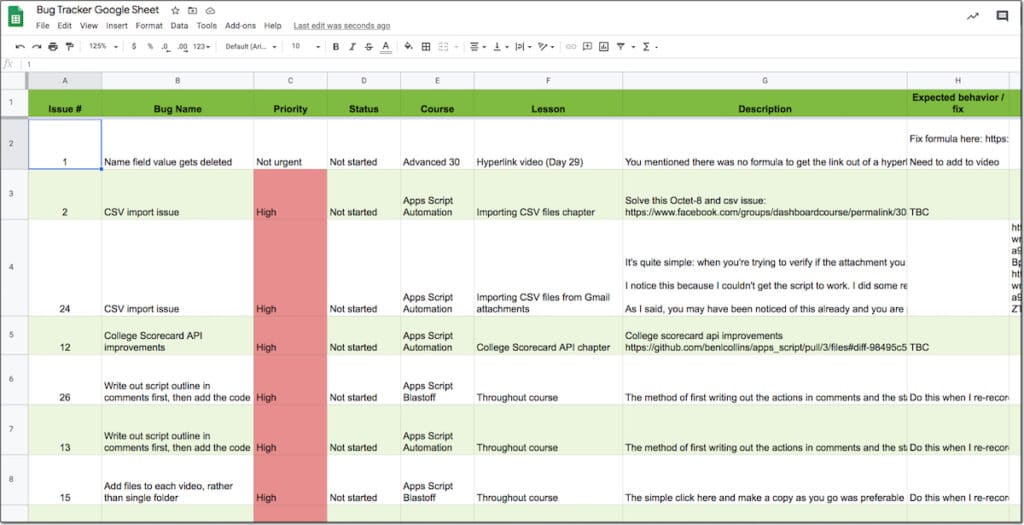And here is that same tracker in a Google Tables workspace:It’s pre-filtered by course and the information is organized and emphasized with the use of colored tags.

It’s much, much easier to navigate and get a sense of the overall picture.

#### Using Forms To Submit Tickets

Google Tables includes forms to allow users to submit data. These are not the same as G Suite Google Forms, but rather a form builder specific to the Tables product.

I’ve created a Form for my course issue tracker Table.

And now that Google Tables has officially launched, I can include this Form in my online school so students are able to submit tickets directly.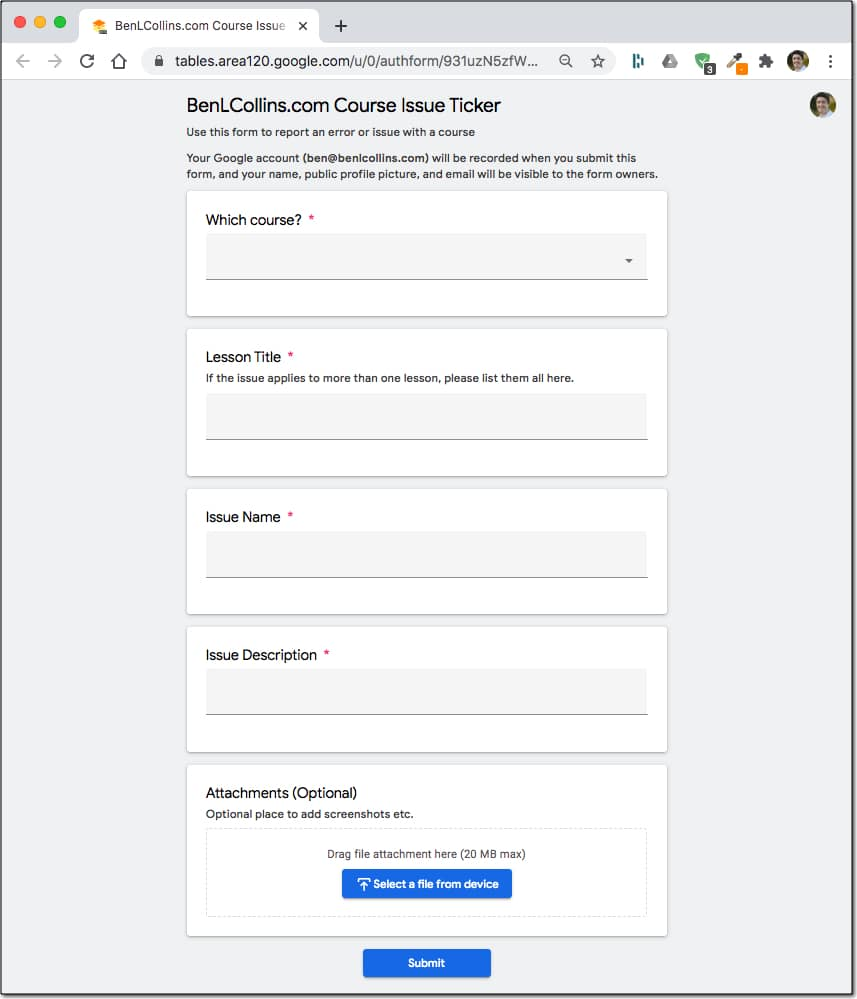### Can I turn my existing Google Sheets into Tables?

Yes! When creating new Tables, you can import data directly from existing Google Sheets.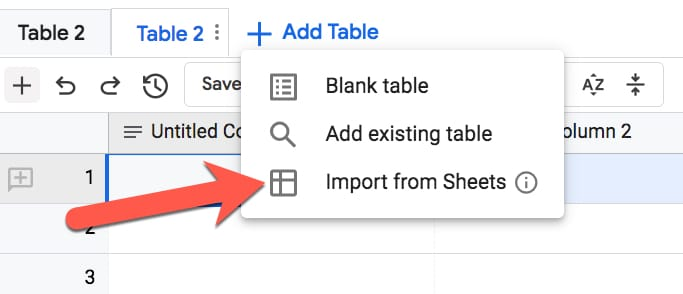### How is Tables different from Google Sheets?

Google Sheets does calculations, summarizes large datasets and creates charts and dashboards. Tables doesn’t do any of those things.

Instead, Google Tables makes it easy to store and organize information, and automate actions. Tables lets you quickly create workflow documents that are easier to use than spreadsheet equivalents.

### Should I move to Google Tables from Trello or Airtable?

The Kanban board layout within Tables is similar to how Trello operates. The bots in Tables allow you to automate tasks in a similar way to Trello’s Butler tool.

Google Tables is similar to Airtable in many ways too. Like Airtable, Google Tables combines some of the best features of spreadsheets with databases, to create an ideal small business workflow and information tool.

Trello and Airtable are more mature products so they do have deeper feature sets, but Tables is new and is bound to develop quickly. Google has deprecated products in the past but I think this is a great tool with enormous potential and I hope Google Tables becomes a major player in this space.

### What Else Can You Do With Google Tables?

Google Tables is designed for businesses, so anytime you’re using spreadsheets for tracking a process, ask if that’s something better suited to Tables.

The Tables team have created a huge number of templates to get you started, everything from a Product Roadmap to an Employee Directory:I plan to share more experiences, tips and use cases for Tables in the coming months.

I’m really excited by this product and see so many opportunities in my own business to improve my existing processes.

## Introduction

Google Sheets custom number format rules are used to specify special formatting rules for numbers.

These custom rules control how numbers are displayed in your Sheet, without changing the number itself. They’re a visual layer added on top of the number. It’s a powerful technique to use because you can combine visual effects without altering your data.

Sheets already has many built-in formats (e.g. accounting, scientific, currency etc.) but you may want to go beyond and create a unique format for your situation.

## Google Sheets Custom Number Format Usage

Access custom number formats through the menu:

Format > Number > More Formats > Custom number format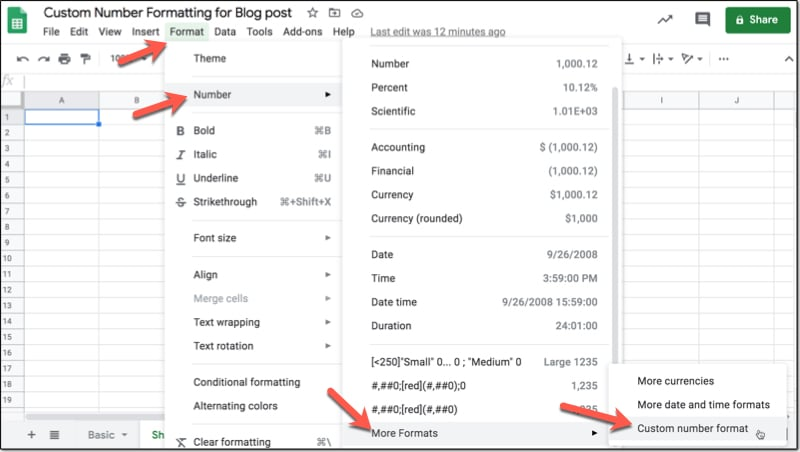The custom number format editor window looks like this: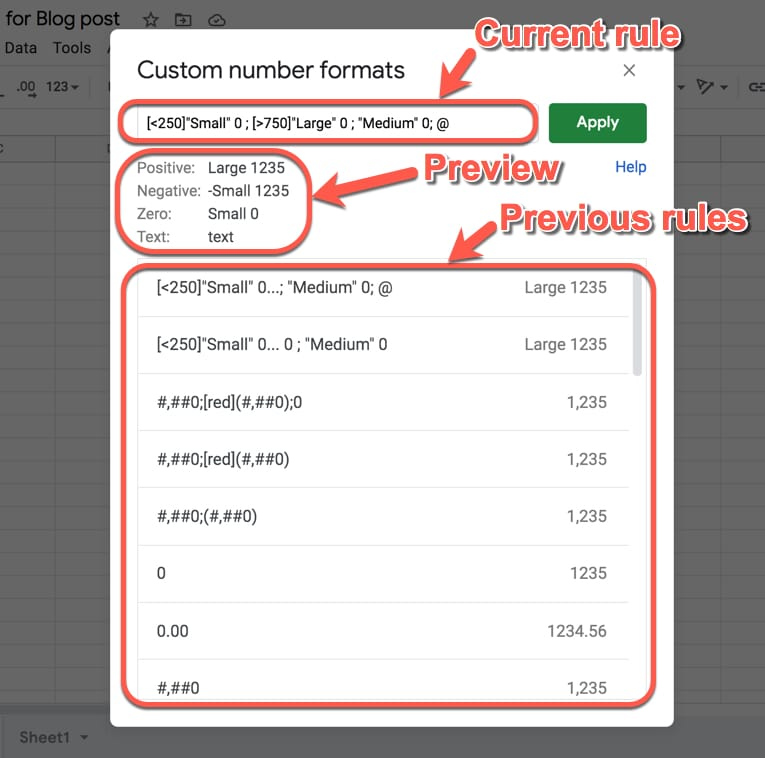You type your rule in the top box and hit “Apply” to apply it to the cell or range you highlighted.

Under the input box you’ll see a preview of what the rule will do. It gives you a useful and pretty accurate indication of what your numbers will look like with this rule applied.

Previous rules are shown under the preview pane. You can click to restore and reuse any of these.

## Google Sheets Custom Number Format Structure

You have four “rules” to play with, which are entered in the following order and separated by a semi-colon:

1. Format for positive numbers
2. Format for negative numbers
3. Format for zeros
4. Format for text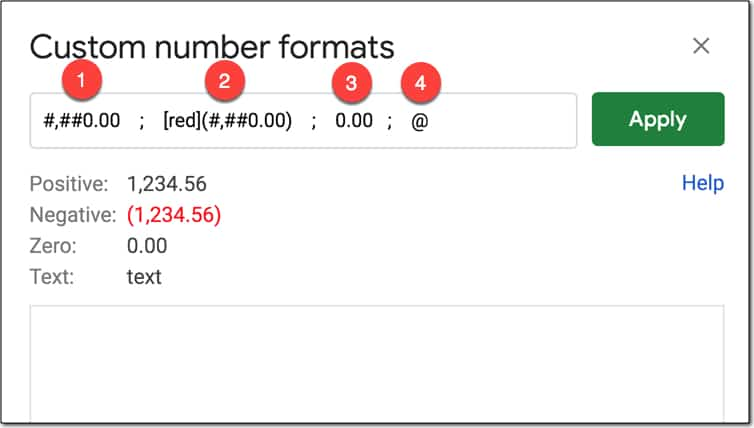### 1. Format for positive numbers

#,##0.00 ; [red](#,##0.00) ; 0.00 ; “some text “@

The first rule, which comes before the first semi-colon (;), tells Google Sheets how to display positive numbers.

### 2. Format for negative numbers

#,##0.00 ; [red](#,##0.00) ; 0.00 ; “some text “@

The second rule, which comes between the first and second semi-colons, tells Google Sheets how to display negative numbers.

### 3. Format for zeros

#,##0.00 ; [red](#,##0.00) ; 0.00 ; “some text “@

The third rule, which comes between the second and third semi-colons, tells Google Sheets how to display zero values.

Rule Before After
`0;0;"Zero"` 0 Zero

### 4. Format for text

#,##0.00 ; [red](#,##0.00) ; 0.00 ; “some text “@

The fourth rule, which comes after the third semi-colon, tells Google Sheets how to display text values.

### Do You Have To Use All Four Rules?

No, you don’t have to specify them all everytime.

If you only specify one rule then it’s applied to all values.

If you specify a positive and negative rule only, any zero value takes on the positive value format.

Here are some examples of single- and multi-rule formats:

Rule Positive Negative Zero Text
`0` 1 -1 0 text
`0;(0)` 1 (1) 0 text
`[red]0` 1 -1 0 text
`0;[red]-0` 1 -1 0 text
`0;[red]-0;[blue]0;[green]@` 1 -1 0 text

## Google Sheets Custom Number Format Rules

### Zero Digit Rule (0)

Zero (0) is used to force the display of a digit or zero, when the number has fewer digits than shown in the format rule. Use the zero digit rule (0) to force numbers to be a certain length and show leading zero(s).

For example:

Rule Before After
`0.00` 1.5 1.50
`00000` 721 00721

### Pound Sign Rule (#)

The pound sign (#) is a placeholder for optional digits. If your value has fewer digits than # symbols in the format rule, the extra # won’t display anything.

Rule Before After
`####` 15 15
`####` 1589 1589
`#.##` 1.5 1.5

### Thousands Separator (,)

The comma (,) is used to add thousand separators to your format rule. The rule `#,##0` will work for thousands and millions numbers.

Rule Before After
`#,##0` 1495 1,495
`#,##0.00` 1234567.89 1,234,567.89

### Period (.)

The period (.) is used to show a decimal point. When you include the period in your format rule, the decimal point will always show, regardless of whether there are any values after the decimal.

Rule Before After
`0.` 10 10.
`0.` 10.1 10.
`0.00` 10 10.00

### Thousands (k or K) or Millions (m or M)

If you add thousand separators but don’t specify a format after the comma (e.g. 0,) then the hundreds will be chopped off the number. Combine this with a “k” or “K” to indicate the thousands and you have a nice way to showcase abbreviated numbers. To achieve this with millions, you need to specify two commas.

Rule Before After
`0.0,` 2500 2.5
`0,"k"` 2500 3k
`0.0,"k"` 2500 2.5k
`0.0,,"M"` 1234567 1.2M

### Negative Number With Brackets ( )

Brackets can be added to the negative number rule to change the format from -100 to (100), which is often seen in accounting and financial scenarios.

Rule Before After
`0;(0)` -100 (100)

### Asterisk (*)

The asterisk (*) is used to repeat digits in your format rule. The character that follows after the asterisk is repeated to fill the width of the cell.

In the following example, the dash is repeated to fill the width of the cell in Google Sheets:

Rule Before After
`*-0` 100 ——————100

### Question Mark (?)

The question mark (?) is used to align values correctly by adding necessary space, even when the number of digits don’t match.

See this example: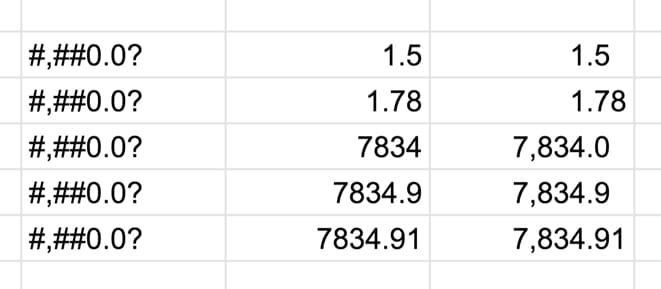### Underscore (_)

In this case, the character that follows the underscore determines the size of the space to add (but is not shown). So this rule allows you to add precise amounts of space.

For example `#,##0.00_);(#,##0.00)` adds a space after the positive sign that is the width of one bracket, so that the decimal point lines up with the negative numbers with brackets.

You can see this clearly in the following image, where the first line does NOT have the spacing but the second line does. The red highlight has been added to show the result of the spacing: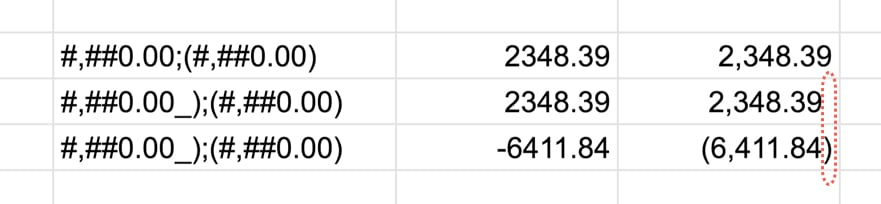### Escape Character (\)

Suppose you want to actually show a pound sign in your format. If you simply add it into your format rule, then Sheets will interpret it as a placeholder for optional digits (see above).

To actually show the pound sign, precede it with a backslash (\) to ensure it shows.

This applies to any of the other special characters too.

Rule Before After
`#0` 10 10
`\#0` 10 #10

### At (@)

The At symbol (@) is used as a placeholder for text, which means don’t change the text entered.

Rule Before After
`0;0;0;"Special text value!"` Some text Special text value!
`0;0;0;@` Some text Some text

### Fraction (/)

The forward slash (/) is used to denote fractions.

For example, the rule `# ?/?` will show numbers as fractions:

Rule Before After
`# ?/?` 2.3333333333 2 1/3

### Percent (%)

The percent sign (%) is used to format values as %. As with the other rules, you first specify the digits and then use the % sign to change to a percent e.g. `0.00%`

Rule Before After
`0.00%` 0.2829 28.29%

### Exponent (E)

For very large (or very small) numbers, use the exponent format rule to show them more compactly.

The rule is: number * E+n, in which E (the exponent) multiplies the preceding number by 10 to the nth power.

Let’s see an example:

Rule Before After
`0.00E+00` 23976986 2.40E+07

## Google Sheets Custom Number Format Conditional Rules

Adding conditions inside of square brackets replaces the default positive, negative and zero rules with conditional expressions.

For example:

Rule Before After
`[<100]"Small" ; [>500]"Large" ; "Medium"` 50 Small
`[<100]"Small" ; [>500]"Large" ; "Medium"` 300 Medium
`[<100]"Small" ; [>500]"Large" ; "Medium"` 800 Large

### Conditional Rules

• Conditions can only be specified in the first two rules
• The third rule is used as the format for everything else that doesn’t satisfy the first two conditions
• The fourth rule is always used for text, so cannot be used for conditional formatting

Meta instructions for conditional rules from the Google Sheets API documentation.

## Colors In Google Sheets Custom Number Formats

There are 8 named colors you can use:
`[black], [white], [red], [green], [blue], [yellow], [magenta], [cyan]`

To get more colors, use the 2-digit color codes written:
`[Color1], [Color2], [Color3], ..., [Color56]`

For full rundown of the color palette for these 56 colors, click here.

### Color Examples

Rule Before After
`0;[red](0)` -100 (100)

Here’s another example of using Google Sheets custom number format rules with colors: How To Make a Table in Google Sheets, and Make It Look Great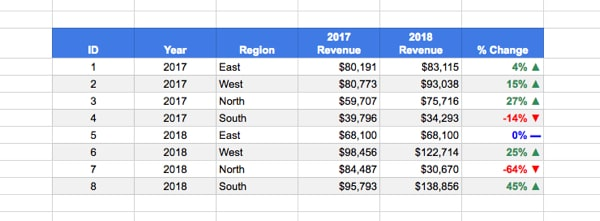where the rule is: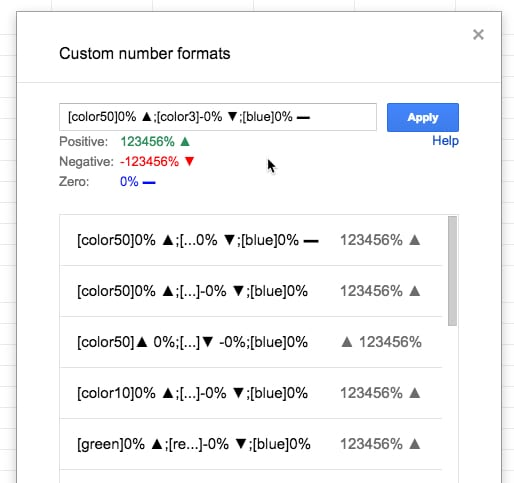Meta instructions for color rules from the Google Sheets API documentation.

## Google Sheets Custom Number Format Examples

### Telephone

Turn any 11 digit number into a formatted telephone number with the zero digit rule and dashes:

Rule Before After
`0 000-000-0000` 18004567891 1 800-456-7891

### Plural

Use conditional rules to pluralize words. Remember, these are still numbers under the hood so you can still do arithmetic with them. The formatting portion (“day” or “days”) is just added as a layer on top.

Rule Before After
`[=1]0" day"; 0" days"` 1 1 day
`[=1]0" day"; 0" days"` 2 2 days
`[=1]0" day"; 0" days"` 100 100 days

### Conditional

Use conditionals to classify numbers directly:

Rule Before After
`[<250]"Small"* 0 ; [>750]"Large"* 0 ; "Medium"* 0` 70 Small 70
`[<250]"Small"* 0 ; [>750]"Large"* 0 ; "Medium"* 0` 656 Medium 656
`[<250]"Small"* 0 ; [>750]"Large"* 0 ; "Medium"* 0` 923 Large 923

Note: these are still numbers under the hood, so you can do arithmetic with them. Moreso, the “Small”, “Medium” and “Large” only exist in the format layer and cannot be accessed in formulas. For example, you can’t use a COUNTIF to count all the values with “Large”. To do that, you need to actually change the value so the word “Large” is in the cell, or add a helper column.

The “* ” part of the rule adds space between the word and the number so that it fills out the full width of the cell.

### Conditional + Color

Add color scales to the conditional example:

Rule Before After
`[color44][<250]"Small"* 0;[color46][>750]"Large"* 0;[color45]"Medium"* 0` 70 Small 70
`[color44][<250]"Small"* 0;[color46][>750]"Large"* 0;[color45]"Medium"* 0` 656 Medium 656
`[color44][<250]"Small"* 0;[color46][>750]"Large"* 0;[color45]"Medium"* 0` 923 Large 923

### Temperature Example

Combine conditionals with emojis to turn numbers into a emoji-scale, like this temperature example:

Rule Before After
`[>90]🔥🔥🔥;[>50]🙂;❄️;"No data"` 37 ❄️
`[>90]🔥🔥🔥;[>50]🙂;❄️;"No data"` 75 🙂
`[>90]🔥🔥🔥;[>50]🙂;❄️;"No data"` 110 🔥🔥🔥
`[>90]🔥🔥🔥;[>50]🙂;❄️;"No data"` N/a “No data”

## Other Resources

Google documentation on how to format numbers in Sheets.

Custom Number Format Builder for Google Sheets and Excel.

## How To Draw The MandelBrot Set In Google Sheets, Using Only Formulas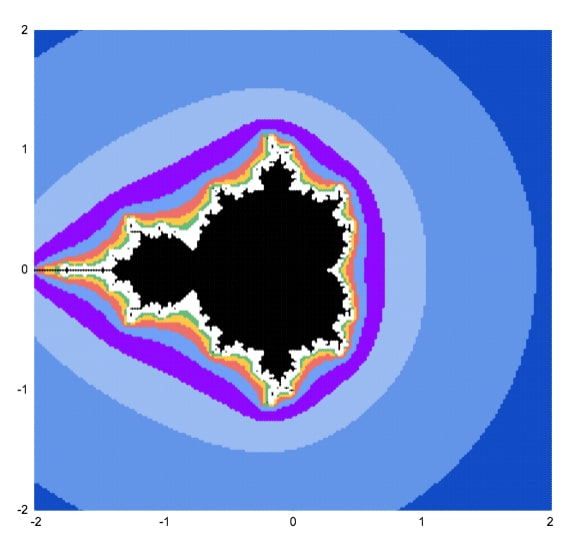This article will walk you through how to draw the MandelBrot set, using only formulas and the built-in chart tool.

## What Is The MandelBrot Set?

The Mandelbrot set is group of special numbers with some astonishing properties.

You’ve almost certainly seen an image of the Mandelbrot set before.

It’s one of the most famous mathematical concepts, known outside of mathematical circles even. It’s named after the French mathematician, Benoit Mandelbrot, who was the father of fractal geometry.

The Mandelbrot set is fantastically beautiful. It’s an exquisite work of art, generated by a simple equation.

More formally, the Mandelbrot set is the set of complex numbers c, for which the equation z² + c does not diverge when iterated from z = 0.

Gosh, what does that mean?

Well, it’s easier if you look at the picture at the top of this article. The black area represents points that do not run away to infinity when you keep applying the equation z² + c.

Consider c = -1.

It repeats -1, 0, -1, 0, -1… forever, so it never escapes. It’s bounded so it belongs in the Mandelbrot set.

Now consider c = 1.

Starting with z = 0, the first iteration is 1.

The second iteration is 1² + 1 = 2.

The third iteration is 2² + 1 = 5.

The fourth iteration is 5² + 1 = 26.

And so on 26, 677, 458330, 210066388901, … It blows up!

It diverges to infinity, so it is not in the Mandelbrot set.

Before we can draw the Mandelbrot set, we need to think about complex numbers.

### Imaginary And Complex Numbers

It’s impossible to draw the Mandelbrot set without a basic understanding of complex numbers.

If you’re new to complex numbers, have a read of this primer article first: Complex Numbers in Google Sheets

It explains what complex numbers are and how you use them in Google Sheets.

To recap, complex numbers are numbers in the form

`a + bi`

where i is the square root of -1.

We can plot them as coordinates on a 2-dimensional plane, where the real coefficient “a” is the x-axis and the imaginary coefficient “b” is on the y-axis.

### Use A Scatter Plot To Draw The Mandelbrot Set

Taking each point on this 2-d plane in turn, we test it to see if it belongs to the Mandelbrot set. If it does belong, it gets one color. If it doesn’t belong, it gets a different color.

This scatter plot chart of colored points is an approximate view of the Mandelbrot set.

As we increase the number of points plotted and the number of iterations we get successively more detailed views of the Mandelbrot set. A point that may still be < 2 on iteration 3 may be clearly outside that boundary by iteration 5, 7 or 10.You can see the outline resembles the Mandelbrot set much more clearly at higher iterations.

## How To Draw The MandelBrot Set In Google Sheets, Using Only Formulas

Here’s a simple approximation of the Mandelbrot set drawn in Google Sheets: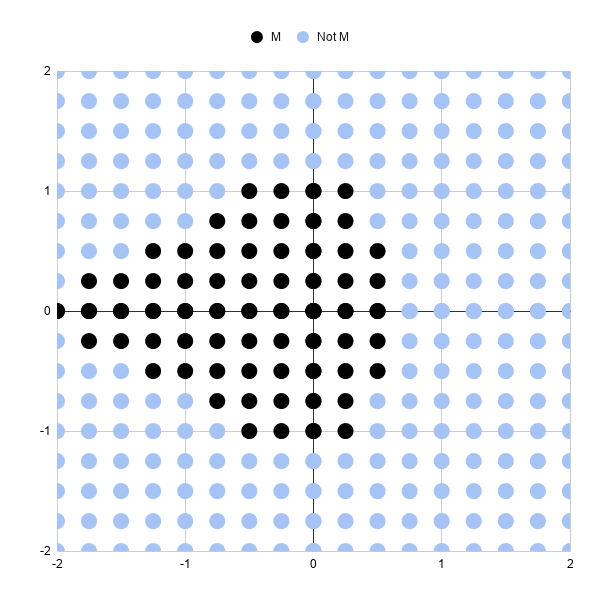Let’s run through the steps to create it.

It’s a scatter plot with 289 points (17 by 17 points).

Each point is colored to show whether it’s in the Mandlebrot set or not, after 3 iterations.

Black points represent complex numbers, which are just coordinates (a,b) remember, whose sizes are still less than or equal to 2 after 3 iterations. So we’re including them in our Mandelbrot set.

Light blue points represent complex numbers whose sizes have grown larger than 2 and are not in our Mandelbrot set. In other words, they’re diverging.

Three iterations is not very many, which is why this chart is a very crude approximation of the Mandelbrot set. Some of these black points will eventually diverge on higher iterations.

And obviously we need more points so we can fill in the gaps between the points and test those complex numbers.

But it’s a start.

### Generating The Complex Number Coordinates

In column A, we need the sequence going from 0 to 2 and repeating { 0, 0.25, 0.5, 0.75, 1, 1.25, 1.5, 1.75, 2, 0, 0.25, 0.5, …}

In column B, we need the sequence 0, then 0.25, then 0.5 repeating 9 times each until we reach 2 { 0, 0, 0, 0, 0, 0, 0, 0, 0, 0.25, 0.25, 0.25, …}

This gives us the combinations of x and y coordinates for our complex numbers c that we’ll test to see if they lie in the set or not.

It’s easier to see in the actual Sheet: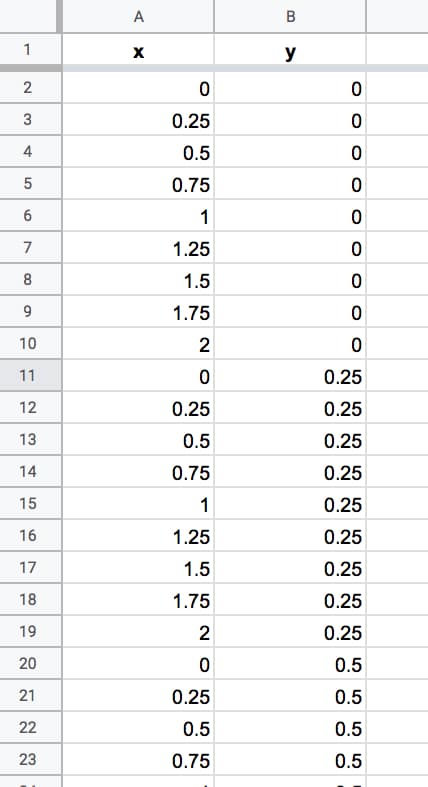We keep going until we reach number 2 in column B. We have 324 rows of data. There are some repeated rows (like 0,0) but that doesn’t matter for now.

Columns A and B now contain the coordinates for our grid of complex numbers. Now we need to test each one to see if it belongs in the Mandelbrot set.

### Formulas For The Mandelbrot Algorithm

We create the algorithm steps in columns C through J.

In cell C2, we create the complex number by combining the real and imaginary coefficients:

`=COMPLEX(A2,B2)`

Cell D2 contains the first iteration of the algorithm with z = 0, so the result is equal to C, the complex number in cell C2, hence:

`=C2`

The second iteration is in cell E2. The equation this time is z² + c, where z is the value in cell D2 and C is the value from C2:

`=IMSUM(IMPOWER(D2,2),\$C2)`

It’s the same z² + c in F2, where z in this case is from E2 (the previous iteration):

`=IMSUM(IMPOWER(E2,2),\$C2)`

Notice the “\$” sign in front of the C in both formulas in columns E and F. This is to lock our formula back to the C value for our Mandelbrot equation.

In cell G2:

`=IMABS(D2)`

Drag this formula across cells H2 and I2 also.

In cell J2:

`=IFERROR(IF(I2<=2,1,2),2)`

Then leave a couple of blank columns, before putting the chart data in columns M, N and O, with the following formulas representing the x values and the two y series with different colors:

`=A2`

`=IF(J2=1,B2,"")`

`=IF(J2=2,B2,"")`

Thus, our dataset now looks like this (click to enlarge):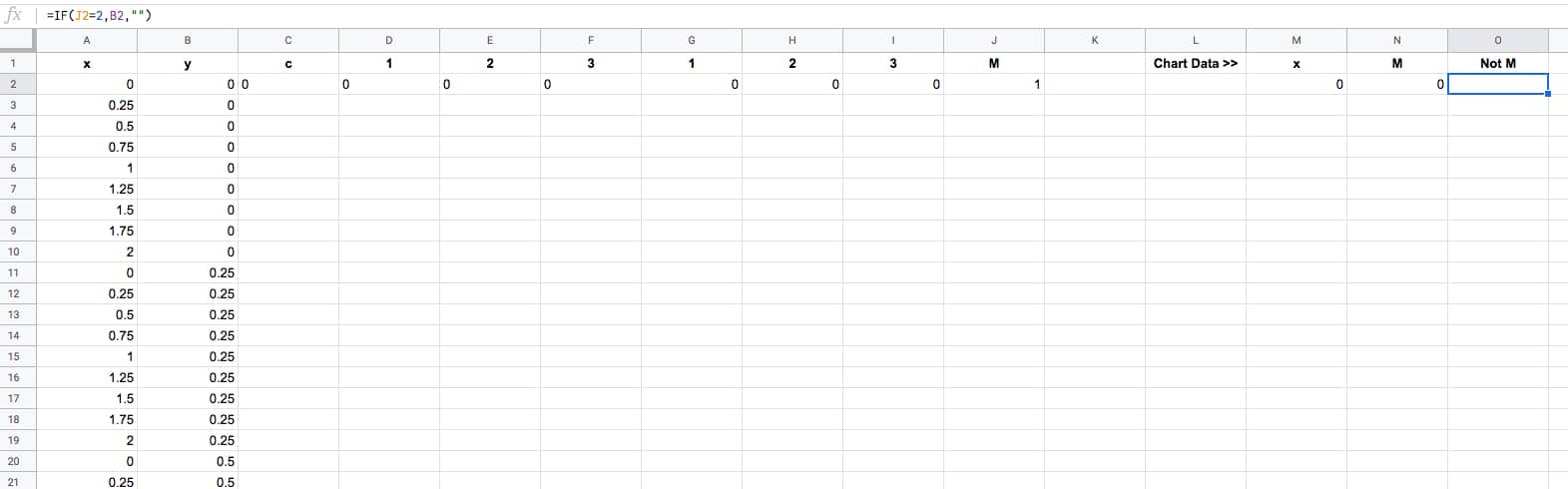The final task is to drag that first row of formulas down to the bottom of the dataset, so that every row is filled in with the formulas (click to enlarge):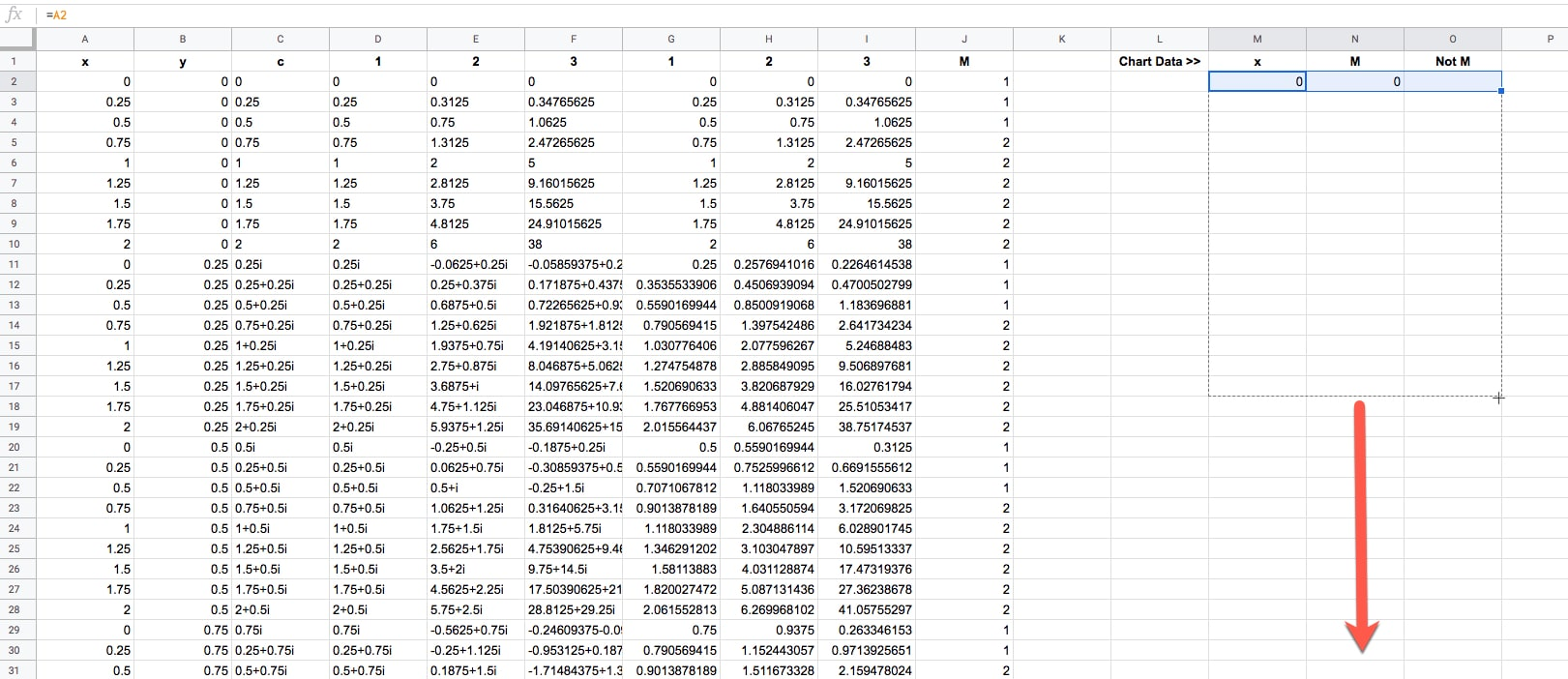Ok, now we're ready to draw some pretty pictures!

### Drawing The Mandelbrot Set In Google Sheets

Highlight columns M, N and O in your dataset.

Click Insert > Chart.

It'll open to a weird looking default Column Chart, so change that to a Scatter chart under Setup > Chart type.

Change the series colors if you want to make the Mandelbrot set black and the non-Mandelbrot set some other color. I chose light blue.

And finally, resize your chart into a square shape, using the black grab handles on the borders of the chart object.

Boom! There it is in all it's glory:Note: you may need to manually set the min and max values of the horizontal and vertical axes to be -2 and 2 respectively in the Customize menu of the chart editor.

## How To Draw A Better MandelBrot Set in Google Sheets

### Generating Data Automatically

Generating those x-y coordinates manually is extremely tedious and not really practical beyond the simple example above.

Thankfully you can use the awesome SEQUENCE function in Google Sheets to help you out. This formula will generate the x-y coordinates for a 33 by 33 grid, which gives a more filled in image than the simple example above.

```=ArrayFormula(UNIQUE({ MOD(SEQUENCE(289,1,0,1),17)/8, ROUNDDOWN(SEQUENCE(289,1,0,1)/17)/8; -MOD(SEQUENCE(289,1,0,1),17)/8, ROUNDDOWN(SEQUENCE(289,1,0,1)/17)/8; MOD(SEQUENCE(289,1,0,1),17)/8, -ROUNDDOWN(SEQUENCE(289,1,0,1)/17)/8; -MOD(SEQUENCE(289,1,0,1),17)/8, -ROUNDDOWN(SEQUENCE(289,1,0,1)/17)/8 }))```

Then drag the other formulas down your rows to complete the dataset, in the same way we did above.

Then you can highlight columns M, N and O to draw your chart again.

Note: you may need to manually set the min and max values of the horizontal and vertical axes to be -2 and 2 respectively in the Customize menu of the chart editor.

With three iterations, the chart looks like: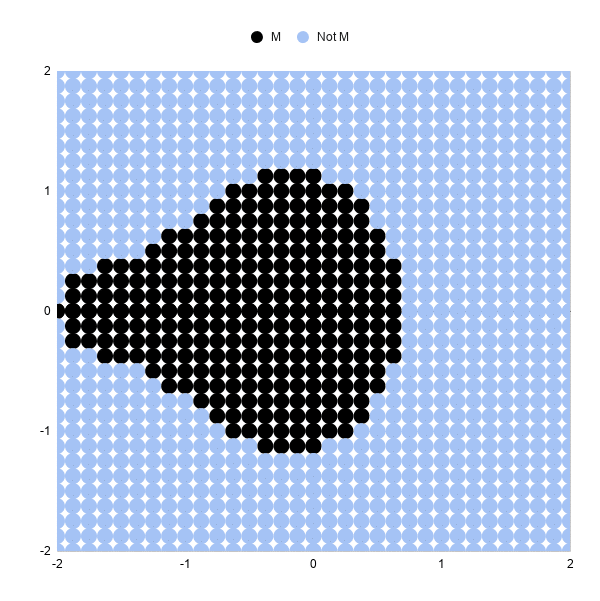### Increasing The Iterations

Let's look at 5 iterations and 10 iterations, and you'll see how much detail this adds.

To move from 3 iterations to 5, we need to add some columns to our Sheet and repeat the algorithm two more times.

So, insert two blank columns between F and G. Label the headings 4 and 5.

Drag the the formula in F2 across the new blank columns G and H (this is the z² + c equation as a Google Sheet formula):

In G2:

`=IMSUM(IMPOWER(F2,2),\$C2)`

In H2:

`=IMSUM(IMPOWER(G2,2),\$C2)`

We need to add the corresponding size calculation columns. Between K and L, insert two new blank columns and drag the IMABS formula across.

Now in L2:

`=IMABS(G2)`

And in M2:

`=IMABS(H2)`

Finally, update the IF statement in column to ensure it's testing the value in column M now:

`=IFERROR(IF(M2<=2,1,2),2)`

And that's it!

Our chart should update and look like this: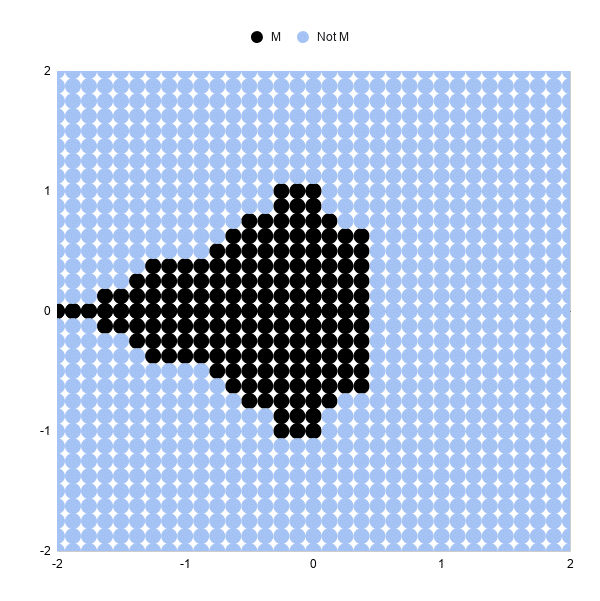You can see the shape of the Mandelbrot set much more clearly now.

Increasing from 5 iterations to 10 iterations is exactly the same. Add 5 blank columns and populate with the formulas again.

The resulting 10 iteration chart is better again: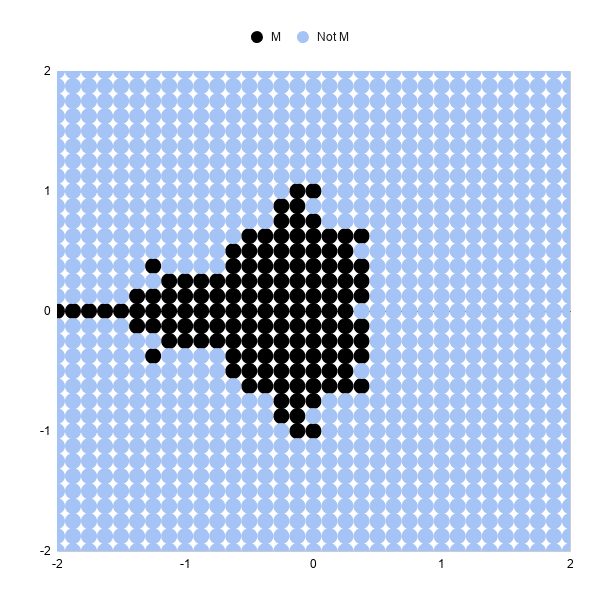### Increasing The Number Of Data Points

Increasing the number of points to 6,561 in an 81 by 81 grid will give a more "complete" picture than the examples above.

This sequence formula will generate these datapoints:

```=ArrayFormula(UNIQUE({ MOD(SEQUENCE(1681,1,0,1),41)/20, ROUNDDOWN(SEQUENCE(1681,1,0,1)/41)/20; -MOD(SEQUENCE(1681,1,0,1),41)/20, ROUNDDOWN(SEQUENCE(1681,1,0,1)/41)/20; MOD(SEQUENCE(1681,1,0,1),41)/20, -ROUNDDOWN(SEQUENCE(1681,1,0,1)/41)/20; -MOD(SEQUENCE(1681,1,0,1),41)/20, -ROUNDDOWN(SEQUENCE(1681,1,0,1)/41)/20 }))```

Be warned, as you increase the number of formulas in your Sheet and the number of points to plot in your scatter chart, your Sheet will start to slow down!

We can add color bands to show which iteration a given point "escaped" towards infinity.

For example, all of the points that are larger than our threshold on iteration 5 get a different color than those that are less than the threshold at iteration 5, but become larger on iteration 6.

The formulas for the iterations and size are the same as the examples above.

Then I determine if the point is still in the Mandelbrot set:

`=IF(ISERROR(AG2),"No",IF(AG2<=2,"Yes","No"))`

And then what iteration it "escapes" to infinity, or beyond the threshold of 2 in this example:

`=IF(AH2="Yes",0,MATCH(2,S2:AG2,1))`

These formulas are shown in the Google Sheets template:

Next we create the series for the chart:

First, the Mandelbrot set:

`=IF(AH2="Yes",B2,"")`

Then series 1 to 15:

`=IF(\$AI2=AK\$1,\$B2,"")`

The range for the scatter plot is then:

`A1:A6562,AJ1:AY6562`

where column A is the x-axis values and columns AJ to AY are the y-axis series.

Drawing the scatter plot and adjusting the series colors results in a pretty picture (this is an 81 by 81 grid):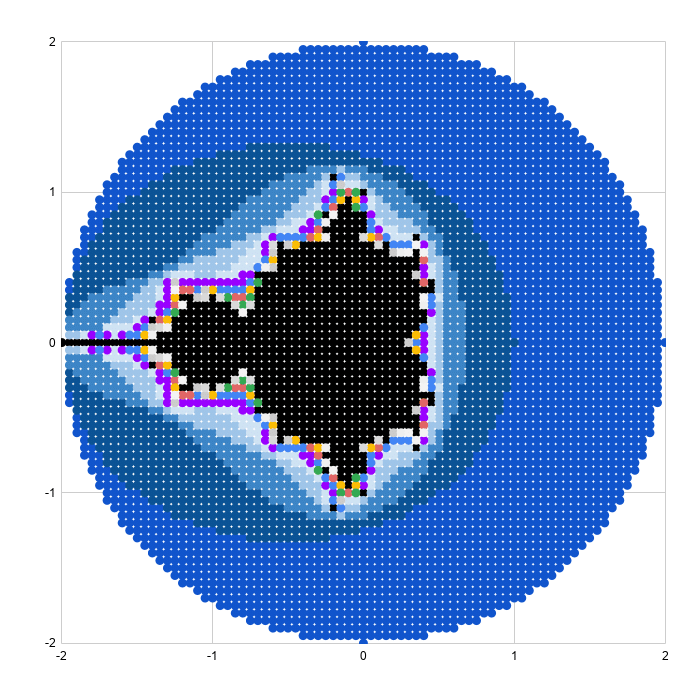### Reaching Google Sheets Practical Limit

As a final exercise, I increased the plot size to 40,401 representing a grid of 201 by 201 points. This really slowed the sheet down and took about half an hour to render the scatter plot, so not something I'd recommend.

The picture is very pretty though!The 40,401 x-y coordinates can be generated with this array formula:

```=ArrayFormula(UNIQUE({ MOD(SEQUENCE(10201,1,0,1),101)/50, ROUNDDOWN(SEQUENCE(10201,1,0,1)/101)/50; -MOD(SEQUENCE(10201,1,0,1),101)/50, ROUNDDOWN(SEQUENCE(10201,1,0,1)/101)/50; MOD(SEQUENCE(10201,1,0,1),101)/50, -ROUNDDOWN(SEQUENCE(10201,1,0,1)/101)/50; -MOD(SEQUENCE(10201,1,0,1),101)/50, -ROUNDDOWN(SEQUENCE(10201,1,0,1)/101)/50 }))```

## Zooming In On The Mandelbrot Set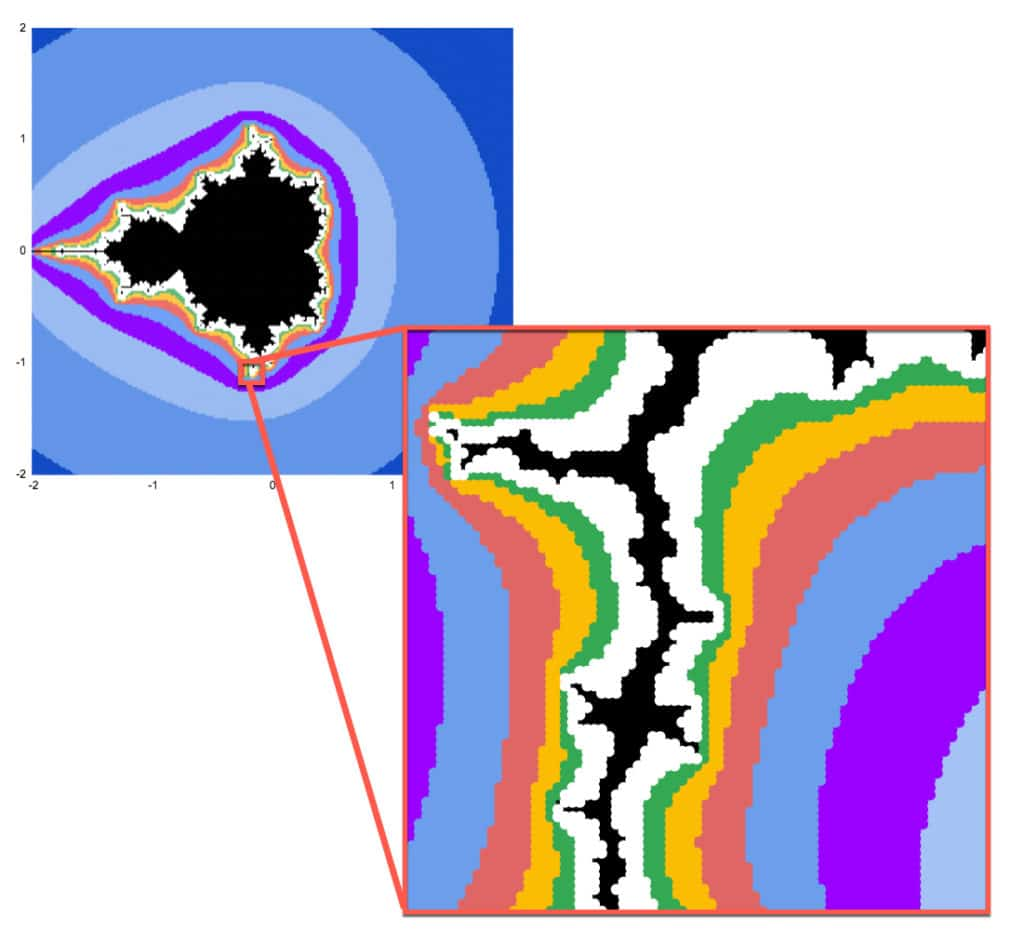Mandelbrot sets have the property of self-similarity.

That is, we can zoom in on any section of the chart and see the same fractal geometry playing out on infinitely smaller scales. This is but one of the astonishing properties of the Mandelbrot set.

Google Sheets is definitely not the best tool for exploring the Mandelbrot set at increasing resolution. It's too slow to render graphically and too manual to make the changes to the formulas and axis bounds.

However, as the maxim says: the best tool is the one you have to hand.

So, if you want to explore in Google Sheets it is possible:

### Generating Zoomed Data

I'm going to zoom in on the point: -0.17033700000, -1.06506000000

(Thanks to this article, The Mandelbrot at a Glance by Paul Bourke, which highlighted some interesting points to explore.)

Starting with this formula in cell A2 to generate the 6,561 data points (I wouldn't recommend going above this because it becomes too slow):

```=ArrayFormula(UNIQUE({ MOD(SEQUENCE(1681,1,0,1),41)/20, ROUNDDOWN(SEQUENCE(1681,1,0,1)/41)/20; -MOD(SEQUENCE(1681,1,0,1),41)/20, ROUNDDOWN(SEQUENCE(1681,1,0,1)/41)/20; MOD(SEQUENCE(1681,1,0,1),41)/20, -ROUNDDOWN(SEQUENCE(1681,1,0,1)/41)/20; -MOD(SEQUENCE(1681,1,0,1),41)/20, -ROUNDDOWN(SEQUENCE(1681,1,0,1)/41)/20 }))```

In columns C and D, I transformed this data by change the 0,0 center to -0.17033700000, -1.06506000000 and then adding the values from A and B to C and D respectively, divided by 100 to zoom in.

`=C\$2+A3/100`
`=D\$2+B3/100`

The rest of the process is identical.

I set the chart axes min and max values to match the min and max values in each of column C (x axis) and D (y axis).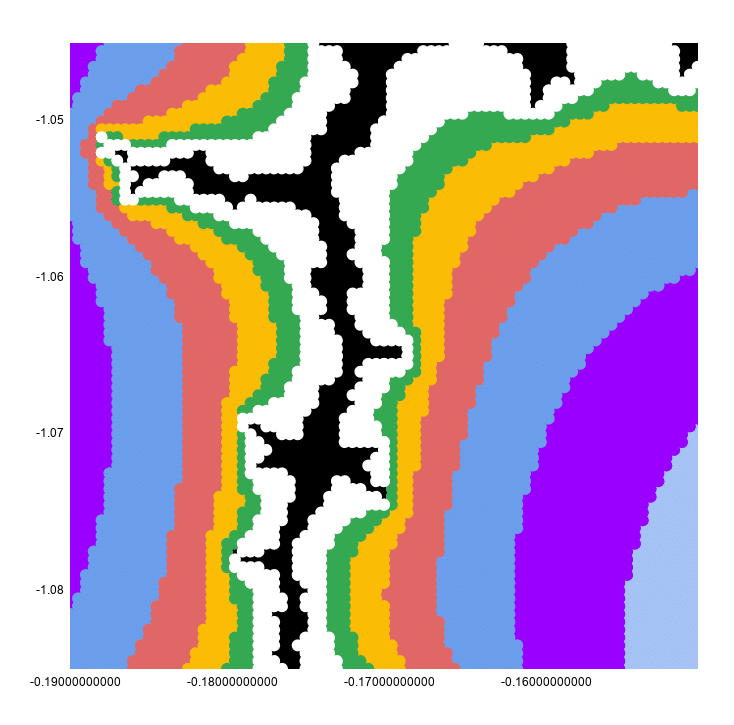This looks continuous because the chart has a point size of 10px to make it look better.

If I reset that to 2px, you can see clearly that this is still a scatter plot: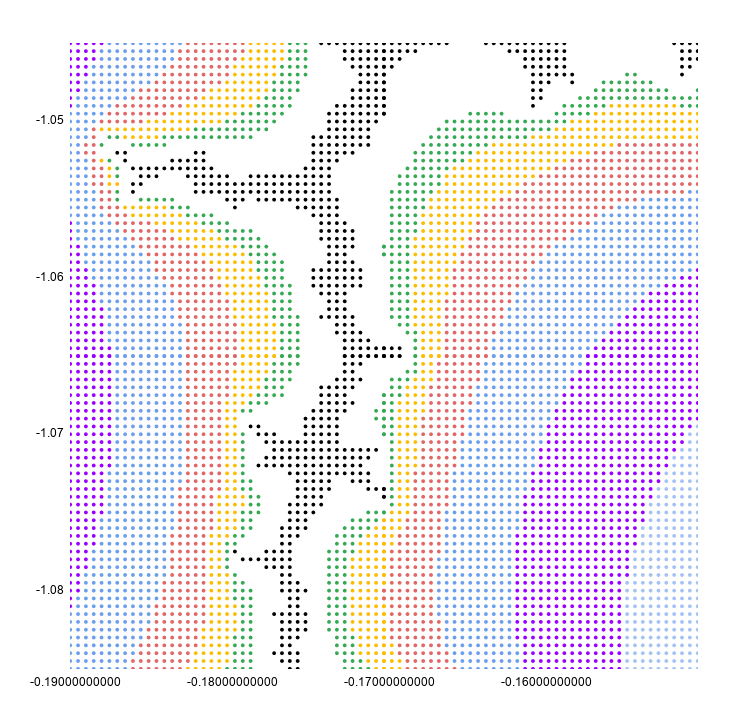I hope you enjoyed that exploration of the Mandelbrot set in Google Sheets! Let me know your thoughts in the comments below.

## Complex Numbers in Google Sheets

Complex numbers are numbers in the form a + bi, where i is the square root of -1.

What on earth does that mean?

## Imaginary And Complex Numbers

To start, consider an integer, say the number 4. The square root of 4 is 2. Easy peasy.

Now consider -4. What’s the square root of that?

It’s not -2, because -2 * -2 = 4 (a minus multiplied by a minus is a positive in mathematics).

No real number will equal the square root of – 4, so we need a new number.

This new number is called an imaginary number (no, I didn’t just make that up!) and is denoted by i and defined as the square root of -1.

`i = √-1`

or put another way:

`i² = -1`

A complex number is a number that has real and imaginary parts, and can be expressed in the form:

`a + bi`

where a and b are real numbers and i is the imaginary number defined above.

We can look at complex numbers visually in a 2-dimensional plane, with the x-axis representing the real numbers and the y-axis representing the imaginary numbers: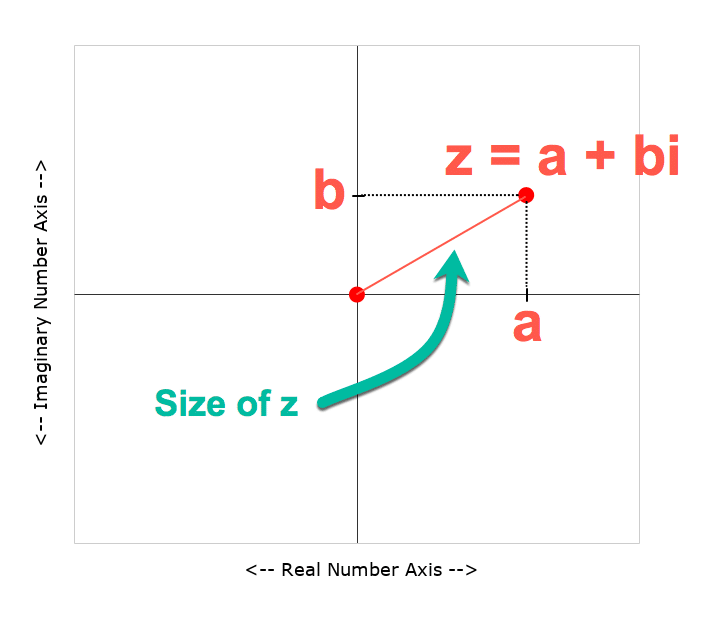Complex numbers are usually represented by the letter z, and written:

`z = a + bi`

Let’s go and create one in Google Sheets!

## Complex Numbers In Google Sheets

Let’s create a complex number in Google Sheets.

Starting in a blank Sheet, add the numbers 3 and 2 into cells A1 and B1 respectively.

In C1, add this formula with the COMPLEX function:

`=COMPLEX(A1,B1,"i")`

This is what our complex number looks like in Google Sheets: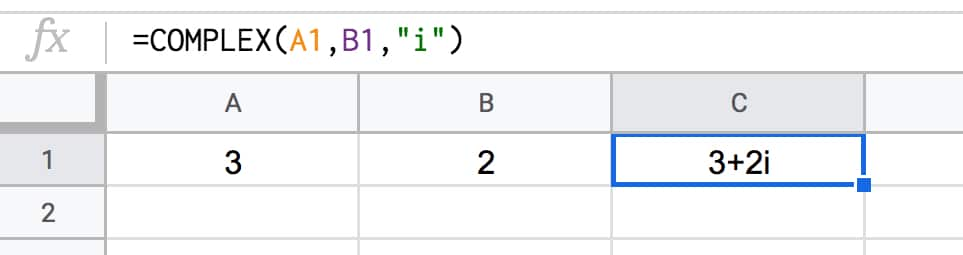### Size Of A Complex Number

We can calculate the size of a complex number, called its absolute value or modulus value and written |z|, by measuring how far it is from the center point (0,0).

We do this using the Pythagorean formula for the longest side of a right angle triangle, namely the square root of the squares of the other two sides.

Google Sheets has a handy function, called IMABS, that will calculate this value for us.

Put this formula into cell D1:

`=IMABS(C1)`

It gives the result 3.605551275.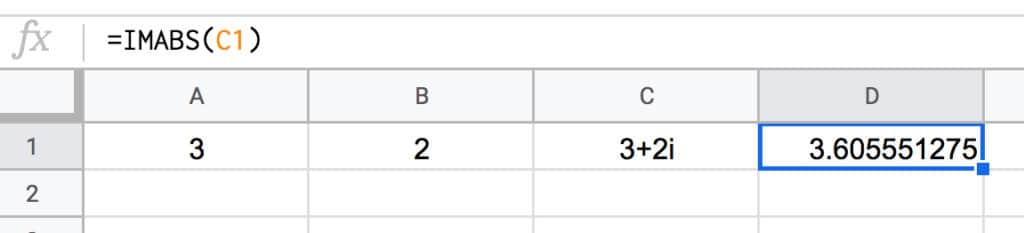This is the same as doing the square root of the squares of the other two sides:

`=SQRT(3^2+2^2)`

The next operation we need to know how to do with complex numbers is how to add them.

What’s 3 + 2i added to 5 + 7i?

To get the answer we add the real parts and imaginary parts separately, and combine into a new complex number.

So

```z = (3 + 2i) + (2 + 7i) = (3 + 2) + (2i + 7i) = 5 + 9i```

Back in our Google Sheet, I’ve added a new row for the new complex number and used the IMSUM function to add them together:

`=IMSUM(C1:C2)`

which looks like this in our Google Sheet: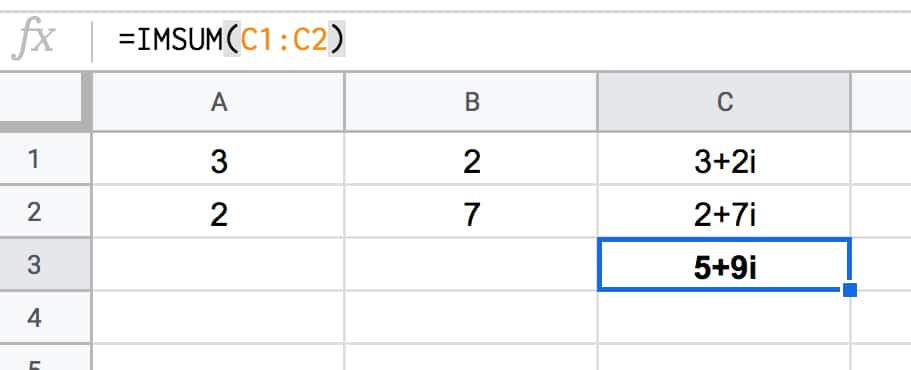### Squaring Complex Numbers

Another operation we need to perform on our complex numbers is to square them.

The IMPOWER function does the trick. It returns a complex number raised to a power.

In our Google Sheet, use this formula in cell D1:

`=IMPOWER(C1,2)`

which gives the answer 5 + 12i, calculated as follows:

```(3 + 2i)² = (3 + 2i) * (3 + 2i) = 3 * 3 + 2i * 3 + 3 * 2i + 2i * 2i = 9 + 6i + 6i + 4 * i² = 9 + 12i + 4 * -1 = 9 - 4 + 12i = 5 + 12i```

### Extracting Real And Imaginary Parts

You can use the IMREAL function to return the real coefficient of a complex number (the “a” part).

And IMAGINARY function returns the imaginary coefficient (the “b” part).

There are other, more specialized complex numbers functions in Google Sheets too, but they’re beyond the scope of this article.

## Why Are Complex Numbers Useful?

For mathematicians, complex numbers are just as “real” as the real numbers.

They have applications in many areas of mathematics, physics and engineering.

One area where you may have seen them without realizing, is in fractal geometry and specifically pictures of a beautiful set of complex numbers called the Mandelbrot set:## Formula Challenge #4: Sort A Column By Last Name

This Formula Challenge originally appeared as Tip #108 of my weekly Google Sheets Tips newsletter, on 29 June 2020. Sign up so you don’t miss future Formula Challenges!

Find all the Formula Challenges archived here.

## The Challenge: Sort A Column By Last Name

Start with a list of any ten two-word names in column A, like so: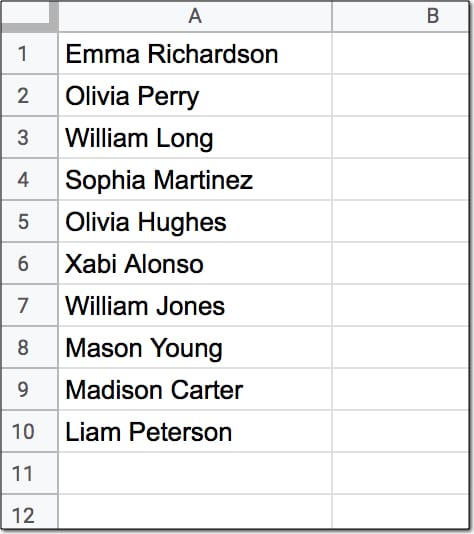Your challenge is to create a single formula in cell B1 (shown in yellow below) that sorts this list alphabetically by the last name, like this: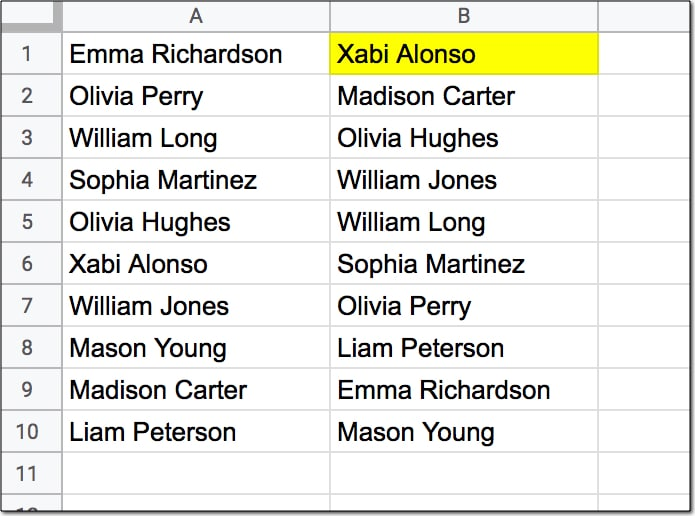Assumptions:

• The names to sort are in the range A1:A10
• Each name consists of a first name and last name only, with a single space between them
• None of the names have prefixes or suffixes
• The formula in cell B1 should output the full names in range B1:B10

## Solutions To Sort A Column By Last Name

I received over 90 replies to this formula challenge with at least 10 different methods for solving it. This was super interesting to see!

Congratulations to everyone who took part.

I learnt so much from the different replies, many of which proffered shorter and more elegant solutions than my own original formula.

Here I present the best 4 solutions, chosen for their simplicity and elegance.

There’s a lot to learn by looking through them.

### 1. SORT/INDEX/SPLIT method

Solution:

Use this formula in cell B1 to sort the data alphabetically by the last name:

`=SORT(A1:A10,INDEX(SPLIT(A1:A10," "),,2),1)`

This is a beautiful solution.

It’s deceptively simple, as you’ll see below when we break it down. And yet, only a handful of people submitted this solution.

How does this formula work?

Let’s use the onion method to peel back the layers and build this formula in steps from the innermost layer.

Step 1:

Enter this formula as a starting point:

`=SPLIT(A1:A10," ")`

It takes the range A1:A10 as an input and splits the names on the space character. That works in this example because of our rigid assumptions that all names are two-word names.

The output is a single row with the name split across two cells. (Adding an ArrayFormula would give an output of all ten names, but it’s not required when we plug this split into the INDEX function.)

Step 2:

Wrap the formula from step 1 in an INDEX function:

`=INDEX(SPLIT(A1:A10," "),,2)`

Leave the row argument (the second argument) in the function blank and choose column 2 in the column argument (the third one). This formula outputs the list of second names, still in the original order however.

Step 3:

The SORT function takes three arguments: the range to sort, the column to sort on, and whether to sort ascending or descending.

So A1:A10, the range containing the full names, is the range we want to sort.

Then the column of last names created in step 2 is the column we want to sort on. This is our second argument.

Then the third argument is a TRUE or 1 to indicate we want to sort the names ascending.

So plugging these three arguments into the SORT function gives the following formula:

`=SORT(A1:A10,INDEX(SPLIT(A1:A10," "),,2),1)`

This formula can be modified to include the whole of column A if there are more than 10 names. Simply change the range from A1:A10 to A1:A as follows:

`=SORT(A1:A,INDEX(SPLIT(A1:A," "),,2),1)`

### 2. QUERY method

Solution:

Use this formula in cell B1 to sort the data alphabetically by the last name:

`=ArrayFormula(QUERY({A1:A10,SPLIT(A1:A10," ")},"select Col1 order by Col3"))`

Step 1:

Step 1 is the same as the step 1 in solution 1 above, and uses the SPLIT to separate the names into first and last names in separate columns:

`=SPLIT(A1:A10," ")`

Step 2:

Step 2 combines these two split columns with the original column of names using curly braces to construct an array of full name, first name and last name.

`=ArrayFormula({A1:A10,SPLIT(A1:A10," ")})`

The ArrayFormula wrapper is necessary to output all 10 values in this example.

Step 3:

The array created by step 2 is used as the input data range in the QUERY function in step 3:

`=ArrayFormula(QUERY({A1:A10,SPLIT(A1:A10," ")},"select Col1 order by Col3"))`

Since the input range of the QUERY function is constructed with the curly brace notation {…} we are required to use the Col1, Col2, etc. notation to access the columns. The query clause is pretty simple. Select only column 1 of the array (the full name) but sort by column 3 (the last name).

Note, the ArrayFormula can be brought outside the QUERY to be the outer wrapper.

This formula can be extended easily to the whole of column A by changing the range to A1:A and adding a WHERE clause to filter out the blank rows:

`=ArrayFormula(QUERY({A1:A,SPLIT(A1:A," ")},"select Col1 where Col1 is not null order by Col3"))`

### 3. REGEX method I

Solution:

Use this formula in cell B1 to sort the data alphabetically by the last name:

`=INDEX(SORT(REGEXEXTRACT(A1:A10,"((.*)( .*))"),3,1),,1)`

If the first solution was the shortest and simplest, then this is perhaps the most elegant.

It only references the data range A1:A10 once, whereas all the other solutions presented here reference the data range twice.

Step 1:

Use the REGEXEXTRACT function to split the data into three columns of full name, first name and last name:

`=REGEXEXTRACT(A1:A10,"((.*)( .*))")`

The REGEX functions in Google Sheets use the re2 flavor of regex.

This formula uses numbered capturing groups to capture the data, denoted by (.*) and ( .*) with the second having a space. The .* simply says match zero or more of any character.

In other words the (.*)( .*) construction separates into first and last names. Adding another matching group with the additional parentheses ((.*)( .*)) also matches the full name.

These three columns are passed into the SORT function in STEP 2:

Step 2:

`=SORT(REGEXEXTRACT(A1:A10,"((.*)( .*))"),3,1)`

The data is sorted on column 3 containing the last name. The 1 represents a TRUE value which sorts the data ascending from A-Z.

Step 3:

In the final step, we use the INDEX function to return only the first column, which has the full name.

`=INDEX(SORT(REGEXEXTRACT(A1:A10,"((.*)( .*))"),3,1),,1)`

### 4. REGEX method II

Solution:

Use this formula in cell B1 to sort the data alphabetically by the last name:

`=SORT(A1:A10,REGEXEXTRACT(A1:A10,"(?: )(\w*)"),1)`

This is the same method as the first solution, but uses REGEXEXTRACT instead of INDEX/SPLIT. So it’s one less function, but the REGEXEXTRACT function is much harder to understand than the INDEX/SPLIT combination for those unfamiliar with the black magic of regular expressions.

Step 1:

Use the REGEXEXTRACT function to extract the last names from the data:

`=REGEXEXTRACT(A1:A10,"(?: )(\w*)")`

The (?: ) is a non-capturing group matching the space. In other words the REGEXEXTRACT matches the space but doesn’t extract it.

The \w character matches word characters (≡ [0-9A-Za-z_]) and the * means match zero or more word characters but prefer more.

The parentheses ( ) around the \w* make it a captured group so it’s extracted.

See, regular expressions are as clear as mud.

Ok, so what’s happening is that it matches on the space, but doesn’t return it. Then it matches on the word group that follows the space and returns that. Hence we get an output range of last names only.

Step 2:

The final step is to sort range A1:A10 using the last names extracted in step 2, and sort them ascending. This step is identical to the implementation in solution 1.

`=SORT(A1:A10,REGEXEXTRACT(A1:A10,"(?: )(\w*)"),1)`

This formula can be modified to include the whole of column A if there are more than 10 names. Simply change the range from A1:A10 to A1:A as follows:

`=SORT(A1:A,REGEXEXTRACT(A1:A,"(?: )(\w*)"),1)`

There we go!

Four brilliant solutions to an interesting formula challenge.